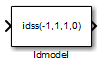# Idmodel

Simulate identified linear model in Simulink software

• Library:
• System Identification Toolbox / Models

•## Description

The Idmodel block simulates the output of an identified linear model using time-domain input data. The model is a state-space (`idss`), linear grey-box (`idgrey`), polynomial (`idpoly`), transfer function (`idtf`), or process (`idproc`) model that you previously estimated or created. For the simulation of state-space and linear grey-box models, you can specify the initial state values. For other linear models, initial conditions are set to zero. You can also add noise to the simulated output.

## Ports

### Input

expand all

Simulation input data, specified as a scalar for a single-input model. The data must be time-domain data. For multi-input models, specify the input as an Nu-element vector, where Nu is the number of inputs. For example, you can use a Vector Concatenate (Simulink) block to concatenate scalar signals into a vector signal.

Note

Do not use a Bus Creator (Simulink) or Mux (Simulink) block to produce the vector signal.

Data Types: `double`

### Output

expand all

Simulated output from linear model, returned as a scalar for a single-output model and an Ny-element vector for a model with Ny outputs.

Data Types: `double`

## Parameters

expand all

Linear model to be simulated, specified as an `idss`, `idgrey`, `idpoly`, `idtf`, or `idproc` object. The model can be continuous-time or discrete-time, with or without input-output delays. You previously estimate or construct the linear model in the MATLAB® workspace.

Initial state values of state-space (`idss`) and linear grey-box (`idgrey`) models, specified as an Nx-element vector, where Nx is the number of states of the model. To estimate the initial states that provide a best fit between measured data and the simulated response of the model for the same input, use the `findstates` command.

For example, to compute initial states such that the response of the model `M` matches the simulated output data in the data set `z`, specify `X0`, such that:

`X0 = findstates(M,z)`

For linear models other than `idss` or `idgrey`, the block assumes that initial conditions are zero.

If you want to reproduce the simulation results that you get in the Model Output plot window in the System Identification app, or from the `compare` command:

1. If the identified model `m` is not a state-space or grey-box model, convert the model into state-space form (`idss` model), and specify the state-space model `mss` in the block.

```mss = idss(m); ```
2. Compute the initial state values that produce the best fit between the model output and the measured output signal using `findstates`. Specify the prediction horizon as `Inf`, that is, minimize the simulation error.

`X0 = findstates(mss,z,Inf);`
3. Use the model `mss` and initial states `X0` in the Idmodel block to perform the simulation. Specify the same input signal `z` for simulation that you used as validation data in the app or `compare`.

When you select this parameter, the block derives the noise amplitude from the linear model property `model.NoiseVariance`. The software filters random Gaussian white noise with the noise transfer function of the model and adds the resulting noise to the simulated model response. If you want to add the same noise every time you run the Simulink® model, specify the `Noise seed(s)` property.

For continuous-time models, the ideal variance of the noise term is infinite. In reality, you see a band-limited noise that accounts for the time constants of the system. You can interpret the resulting simulated output as filtered using a lowpass filter with a passband that does not distort the dynamics from the input.

The Noise seed(s) property seeds the random number generator such that the block adds the same noise to the simulated output every time you run the Simulink model. For information about using seeds, see `rng`.

For multi-output models, you can use independent noise realizations that generate the outputs with additive noise. Enter a vector of Ny nonnegative integer entries, where Ny is the number of output channels.

For random restarts that vary from one simulation to another, specify Noise seed(s) as `[]`.

#### Dependency

To enable this parameter, select ```Add noise```.

## Version History

Introduced in R2008a